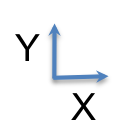Rubik's Cube !

Player-Specified Rotations

Rotations

Rubik's Cube has 54 (small) visible squares: 9 on each of the 6 faces of the Cube. The Player rotates different "slices" to try to get a single color on each face. We place the Cube in an XYZ coordinate system with one vertex at the Origin (0,0,0) and the opposite vertex at (2,2,2). The 6 faces coincide with the planes X=0, Y=0, Z=0, X=2, Y=2, and Z=2. Click "Random Shuffle" to create a Starting position. By then clicking in sequence any of 18 possible individual rotations, the Player's goal is to return all 54 small squares to the "Solved" positions.

Order Parameter

Six Faces

#1: X = 0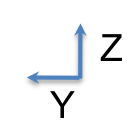#2: Y = 0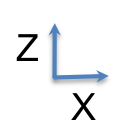#3: Z = 0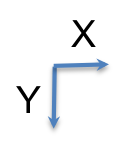#4: X = 2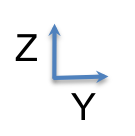#5: Y = 2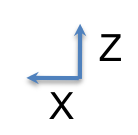#6: Z = 2